# Model a Planar Pendulum

Consider a point mass m suspended by a massless rod of length l under the influence of gravity. The position of the mass can be expressed in Cartesian coordinates by (x,y).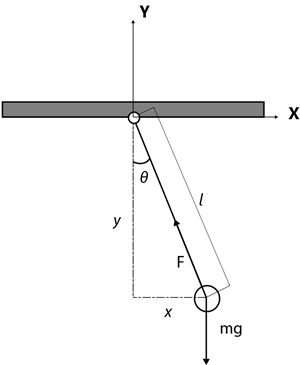### Modeling the System

A force balance of the mass gives the equations of motion in the x and y directions.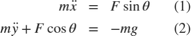Let (u, v) be the velocities in (x, y) respectively. The system can be rewritten as a system of first order ODEs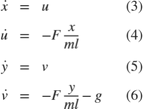where F is the tension in the rod. The system also possesses the geometric constraint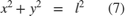Differentiate (7) twice with respect to time t to arrive at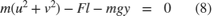This relationship is useful since it allows F to determined at every step for use in modeling the kinematics of the system.

### Simulating the System

The system is simulated as shown in the figure below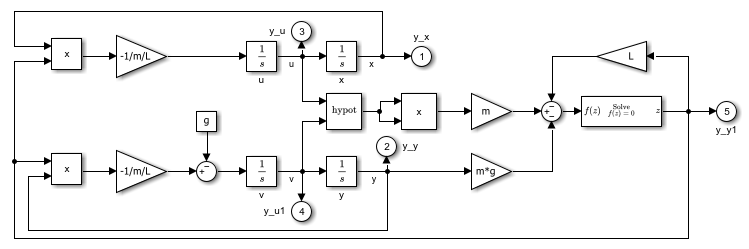Equation (8) contains one unknown F and is of the form f(z) = 0 where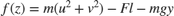. The Algebraic Constraint block constrains f(z) to 0 and solves for F in accordance with (8).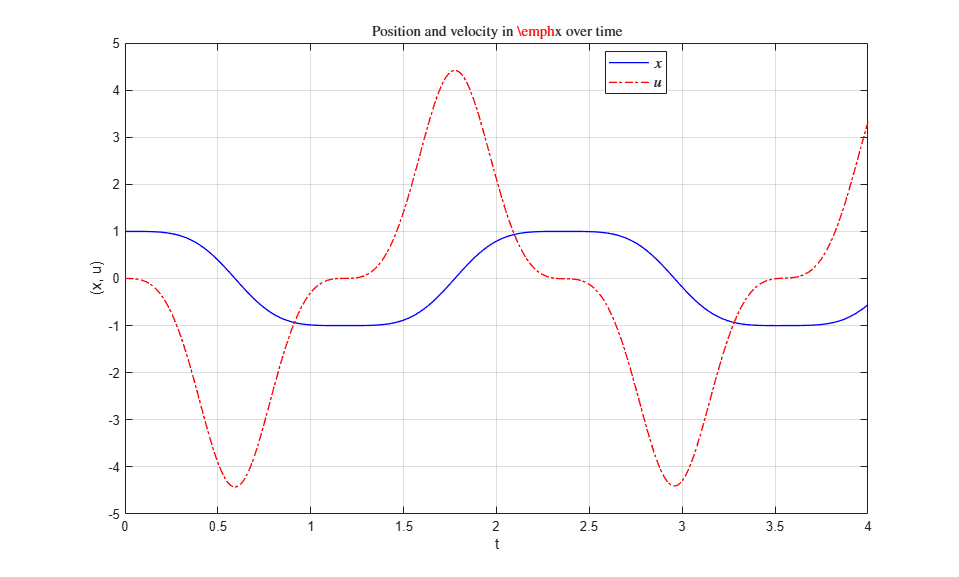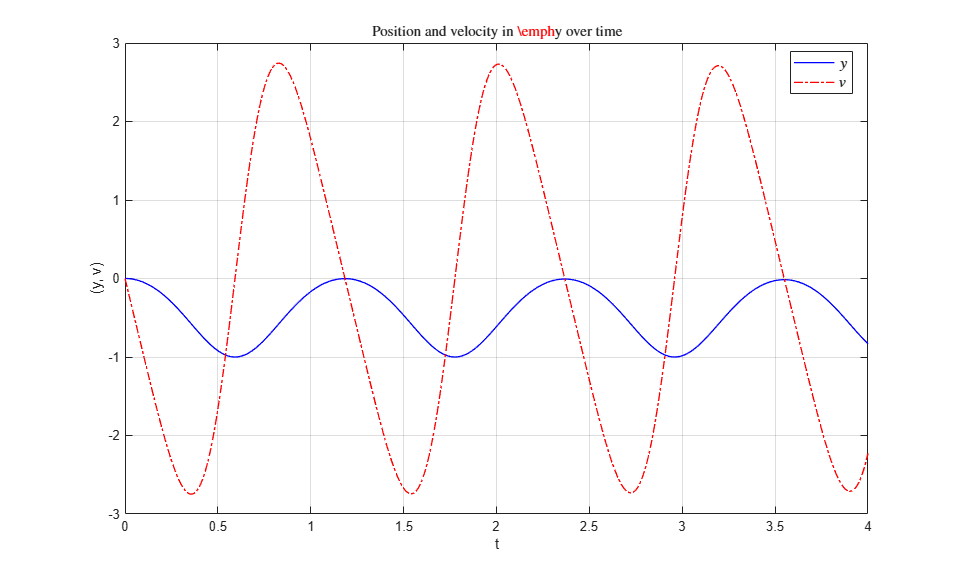### References

Hairer, Ernst, Christian Lubich, and Michel Roche. "The Numerical Solution Of Differential-Algebraic Systems By Runge-Kutta Methods." Lecture Notes in Mathematics. Vol. 1409, Berlin: Springer-Verlag, 1989: pp. 8-9.